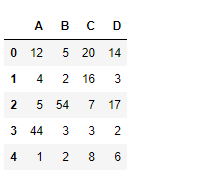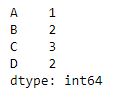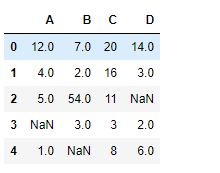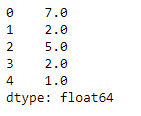Open in App
Not now

# Python | Pandas dataframe.min()

• Last Updated : 24 Nov, 2018

Python is a great language for doing data analysis, primarily because of the fantastic ecosystem of data-centric python packages. Pandas is one of those packages and makes importing and analyzing data much easier.

Pandas` dataframe.min()` function returns the minimum of the values in the given object. If the input is a series, the method will return a scalar which will be the minimum of the values in the series. If the input is a dataframe, then the method will return a series with minimum of values over the specified axis in the dataframe. By default the axis is the index axis.

Syntax:DataFrame.min(axis=None, skipna=None, level=None, numeric_only=None, **kwargs)
Parameters :
axis : Align object with threshold along the given axis.
skipna : Exclude NA/null values when computing the result
level : If the axis is a MultiIndex (hierarchical), count along a particular level, collapsing into a Series
numeric_only : Include only float, int, boolean columns. If None, will attempt to use everything, then use only numeric data. Not implemented for
Series.

Returns : min : Series or DataFrame (if level specified)

Example #1: Use `min()` function to find the minimum value over the index axis.

 `# importing pandas as pd ` `import` `pandas as pd ` ` `  `# Creating the dataframe  ` `df ``=` `pd.DataFrame({``"A"``:[``12``, ``4``, ``5``, ``44``, ``1``], ` `                   ``"B"``:[``5``, ``2``, ``54``, ``3``, ``2``],  ` `                   ``"C"``:[``20``, ``16``, ``7``, ``3``, ``8``],  ` `                   ``"D"``:[``14``, ``3``, ``17``, ``2``, ``6``]}) ` ` `  `# Print the dataframe ` `df `Lets use the `dataframe.min()` function to find the minimum value over the index axis

 `# find min Even if we do not specify axis = 0, the method  ` `# will return the min over the index axis by default ` `df.``min``(axis ``=` `0``) `

Output :Example #2: Use `min()` function on a dataframe which has `Na` values. Also find the minimum over the column axis.

 `# importing pandas as pd ` `import` `pandas as pd ` ` `  `# Creating the dataframe  ` `df ``=` `pd.DataFrame({``"A"``:[``12``, ``4``, ``5``, ``None``, ``1``], ` `                   ``"B"``:[``7``, ``2``, ``54``, ``3``, ``None``], ` `                   ``"C"``:[``20``, ``16``, ``11``, ``3``, ``8``],  ` `                   ``"D"``:[``14``, ``3``, ``None``, ``2``, ``6``]}) ` ` `  `# Print the dataframe ` `df `Lets implement the min function.

 `# skip the Na values while finding the minimum ` `df.``min``(axis ``=` `1``, skipna ``=` `True``) `

Output :My Personal Notes arrow_drop_up
Related Articles